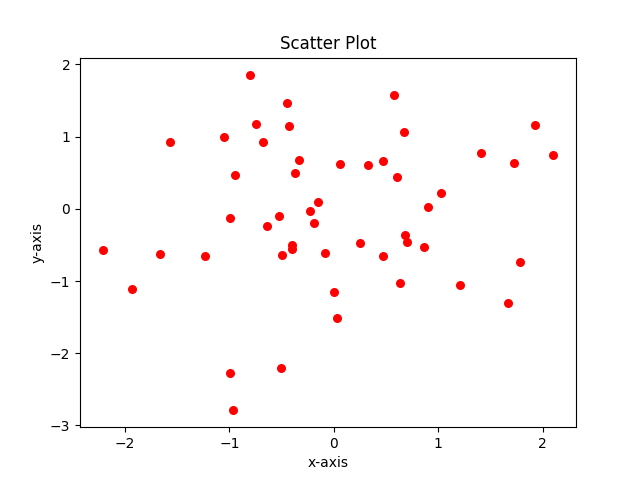Related Tags

matplotlib
python
scatter
chart

# How to create a scatter chart using matplotlibEducative Answers Team

A scatter plot is a type of plot that shows data as a collection of points. A point’s position depends on its two-dimensional value, where each value is positioned on either the horizontal or vertical dimension.Matplotlib has a built-in function to create scatterplots called scatter().

## Syntax

Take a look at the syntax below:

matplotlib.pyplot.scatter(x, y, s=None, c=None, marker=None, ...)


x and y are the data points on the x and y-axis. The function also has several other optional parameters such as color, area, alpha values, etc. Click here for further details.

## Code

The example below outputs a scatter plot that consists of randomly generated data points:

import numpy as np
import matplotlib.pyplot as plt

num = 50
x = np.random.rand(num)
y = np.random.rand(num)

# alpha value denotes the transparency. higher the value, more opaque the element is, visually
plt.scatter(x, y, c='red', alpha=0.8)
plt.title('Scatter plot')
plt.xlabel('x-axis')
plt.ylabel('y-axis')
plt.show()

RELATED TAGS

matplotlib
python
scatter
chart